# Lexicographic order

(diff) ← Older revision | Latest revision (diff) | Newer revision → (diff)

An order on a direct product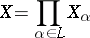of partially ordered sets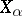(cf. Partially ordered set), where the set of indicesis well-ordered (cf. Totally well-ordered set), defined as follows: If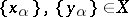, thenif and only if eitherfor all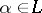or there is ansuch that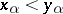and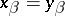for all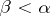. A setordered by the lexicographic order is called the lexicographic, or ordinal, product of the sets. If all the setscoincide (for all), then their lexicographic product is called an ordinal power ofand is denoted by. One also says thatis ordered by the principle of first difference (as words are ordered in a dictionary). Thus, ifis the series of natural numbers, then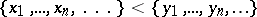means that, for some,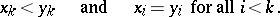The lexicographic order is a special case of an ordered product of partially ordered sets (see ). The lexicographic order can be defined similarly for any partially ordered set of indices(see ), but in this case the relation on the set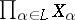is not necessarily an order in the usual sense (cf. Order (on a set)).

A lexicographic product of finitely many well-ordered sets is well-ordered. A lexicographic product of chains is a chain.

For a finite, the lexicographic order was first considered by G. Cantor

in the definition of a product of order types of totally ordered sets.

The lexicographic order is widely used outside mathematics, for example in ordering words in dictionaries, reference books, etc.

How to Cite This Entry:
Lexicographic order. Encyclopedia of Mathematics. URL: http://encyclopediaofmath.org/index.php?title=Lexicographic_order&oldid=13984
This article was adapted from an original article by T.S. Fofanova (originator), which appeared in Encyclopedia of Mathematics - ISBN 1402006098. See original article